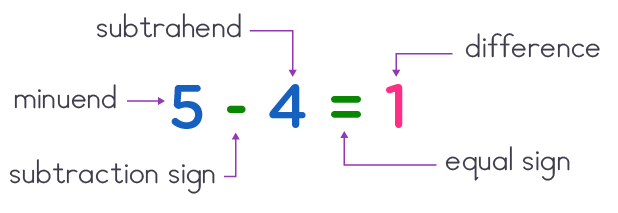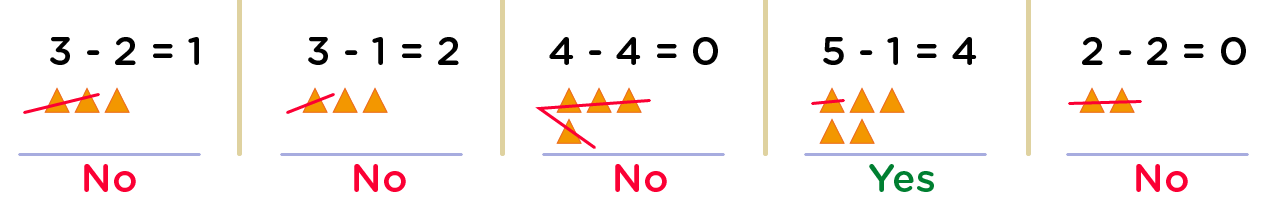# Kindergarten math: Subtracting within 5 worksheets

Hello, and welcome to this fun kindergarten math resource! We are excited to share some fantastic subtraction worksheets that will help your kindergarten students learn how to perform subtraction within 5 efficiently.

Subtraction is a key skill that will prepare young math learners for more advanced math concepts in the future. Hence grasp these excellent worksheets from MathSkills4kids, designed in a fun, colorful, and engaging way to make your kids love math!

• ## How to teach your kids to subtract within 5 in a fun way

One way to teach your kids how to subtract within 5 is by using fun ideas that stimulate their interest to learn. But before then, let's recall what subtraction is.

Hello, math lovers! Are you ready to learn about subtraction? Subtraction is one of the basic operations in math, and it means taking away some amount from another amount.

For example, if you have 5 apples and eat 2 of them, you have 5 - 2 = 3 apples left.

From the above example, you see that subtraction is essential because it helps us determine how much is left or how much more we need.

That said, we will show you some fun and easy ways to practice subtracting within 5 using pictures and objects. We will also give you some worksheets you can print and use at home or in the classroom. Let's get started!

• ### What are the parts of a subtraction sentence?

For your kinders to become math wizards and proficient in subtraction in particular, simply grab our free printable subtraction math for kindergarten. This worksheet will instill excitement into kinders math lives as they will tackle subtraction problems only with basic numbers up to 5.

Before moving on to solving subtraction sentences in our subtraction for kindergarten pdf, it is very important for kids to have an in-depth understanding of parts of a subtraction sentence.

Parts of a subtraction sentence include• The subtrahend, which is the number to be taken away, and
• The difference, which is the part left after subtracting. It comes after the equal sign (=)
• Equally important is the subtraction sign, also referred to as “take away” or “minus”. It’s represented thus “

An example of a subtraction sentence is →         2      -     1    =    1

• ### How to use pictures and objects to subtract within 5

One of the best ways to learn subtraction within 5 is to use pictures and objects that you can see and touch. For example, you can use crayons, buttons, stickers, or anything else that you have around. Here are some steps to follow:

• First, choose two numbers that are less than or equal to 5. For example, let's choose 4 and 2.
• Next, draw or place 4 objects on a paper or a table. These are the total number of objects that you start with.
• Then, draw a line under the objects and write the number 4 below the line. This is the first number in the subtraction equation.
• After that, cross out or take away 2 objects from the group. These are the objects that you are subtracting.
• Finally, count how many objects are left and write the number below the line. This is the second number in the subtraction equation and the answer to the problem. For example, 4 - 2 = 2.

Repeat this process with different numbers and objects until you feel comfortable subtracting within 5.

• ### Subtracting from 5: fill in the missing number

Another way to practice subtracting within 5 is to fill in the missing number in a subtraction equation.

For example, if you see 5 - __ = 3, you must figure out what number goes in the blank space.

To do this, you can use pictures and objects as we did before, or you can use your fingers. Here are some steps to follow:

• First, hold up 5 fingers on one hand. These represent the total number of objects that you start with.
• Next, look at the number after the minus sign in the equation. This is the number of objects that you are subtracting. For example, in 5 - __ = 3, the number after the minus sign is missing, so we still need to know how many objects we are subtracting.
• Then, look at the number at the end of the equation. This is the number of objects that are left after subtracting. For example, in 5 - __ = 3, the number at the end of the equation is 3, so we know that there are 3 objects left after subtracting.
• Finally, fold down or hide as many fingers as needed to match the number at the end of the equation. The number of fingers that are still up is the missing number in the equation. For example, in 5 - __ = 3, we need to fold down or hide 2 fingers to match 3 fingers left. The number of fingers that are still up is 2, so we can fill in the blank space with 2.
• ### How to solve real-life situations involving subtraction within 5

Do you want your kids to become creative thinkers at this early stage? If yes! then grasp this resource and teach them how to think and solve real-life situations involving subtraction within 5 using simple and fun strategies.

Subtraction is not only beneficial for math problems but also for real-life situations.

For example, you can use subtraction to find out how many cookies are left after sharing with your friends, how many minutes are left until your favorite show starts, or how much money you have left after buying something.

#### Here is an example of a real-life situation involving subtraction within 5:

You have \$5 in your pocket, and you want to buy a candy bar that costs \$2. How much money will you have left after buying the candy bar?

To solve this problem, you can use pictures and objects or your fingers as we did before. You can also use mental math or a calculator if you prefer.

• One way to solve this problem is to use pictures and objects. You can draw or place 5 coins on paper or a table. These represent the total amount of money that you start with.
• Then, draw a line under the coins and write \$5 below the line. This is the first number in the subtraction equation.
• After that, cross out or take away 2 coins from the group. These represent the cost of the candy bar that you are subtracting.
• Finally, count how many coins are left and write \$ below them. This is the second number in the subtraction equation and how much money you have left after buying the candy bar. The answer is \$5 - \$2 = \$3.

Repeat this process with real-life situations until you feel comfortable applying subtraction within 5.

• ### Tips and tricks to master subtraction within 5

• Start with visual aids: Before diving into subtraction problems, it's essential to introduce visual aids to help children understand the concept of taking away. Use objects such as blocks, toys, or even fingers to demonstrate the process of subtraction.
• Use simple language: When explaining subtraction to kindergarteners, use simple language and avoid using complex terms. Instead of saying "subtract," use phrases like "take away" or "minus."
• Practice counting backward: Counting backward is an essential skill for subtraction.

Encourage children to practice counting backward from 5 to 0 and then move on to subtracting numbers within 5.

• Use manipulatives: Manipulatives such as counting bears or cubes can help children visualize subtraction problems.

Have them start with several objects and then take away a certain amount to find the answer.

• Play games: Make subtraction fun by incorporating games into your lessons. Use flashcards, dice, or a simple board game to practice subtracting within 5.
• Use real-life examples: Help children understand the practical applications of subtraction by using real-life examples. For instance, how many apples are left if there are 5 apples and you eat 2?
• Provide plenty of practice: Repetition is essential when it comes to mastering subtraction within 5. Provide plenty of opportunities for children to practice, whether it's through worksheets, games, or hands-on activities.

### Fun and easy to solve – subtraction sentences: basic numbers up to 5

Subtraction up to 5 worksheets for kindergarten has very fun and easy to solve subtraction exercises. These subtraction sentences: basic numbers up to 5 will enable kids to quickly grasp the rules of subtraction with less stress.

However, by using these our subtraction for kindergarten pdf worksheets, kids will be kept engaged as they find it exciting and anxious to know what is “left”.

Furthermore, in order to gain kid’s attention and concentration, we have well formulated exercises that will recreate kid’s logical thinking progression.

An example of one of such exercises is how do you make 4?   Circle the correct subtraction sentence. Hey kiddos, from a given multiple of subtraction sentences, let’s find out below the method to use to obtain the correct subtraction sentence.

### Example: how do you make 4? Circle the correct subtraction sentence.

3 - 2          3 - 1          4 - 4          5 - 1          2 - 2

Hey kids, as earlier said, we are going to find out which one of the above subtraction sentences can make 4. So, how do you make 4?

• Firstly, we will represent all the minuends in each subtraction sentence with objects.• Next, we’ll now take away by crossing out all the objects that represent the subtrahend in each multiplication sentence
• After crossing all the objects that represent the subtrahend, we can now count the number of uncrossed objects in each case. It is awesome to note that the number of objects uncrossed is the difference.WOW! This is so perfect

• Now, in respect to our question how do you make 4? We’ll look for the subtraction sentence whose difference is 4, then of course circle it.

Oooops! I got it. The correct subtraction sentence is → 5 - 1

BRAVOO! It’s so simple kiddos. Isn’t it? Have fun ok. And continue solving your subtraction problems diligently.

Thank you very much for choosing to share the MathSkills4Kids.com links with your loved ones!